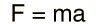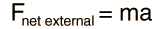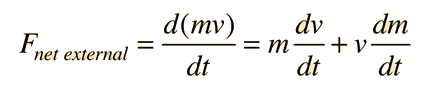Limitations on Newton's 2nd Law

One of the best known relationships in physics is Newton's 2nd Lawbut, though extremely useful, it is not a fundamental principle like the conservation laws. F must be the net external force, and even then a more fundamental relationship isThe net force should be defined as the rate of change of momentum; this becomesonly if the mass is constant. Since the mass changes as the speed approaches the speed of light, F=ma is seen to be strictly a non-relativistic relationship which applies to the acceleration of constant mass objects. Despite these limitations, it is extremely useful for the prediction of motion under these constraints.

Index

Newton's laws concepts

 HyperPhysics***** Mechanics R Nave
Go Back

Limitations on Newton's 2nd Law

One of the best known relationships in physics is Newton's 2nd Lawbut, though extremely useful, it is not a fundamental principle like the conservation laws. F must be the net external force, and even then a more fundamental relationship isThe net force should be defined as the rate of change of momentum; this becomesonly if the mass is constant. Since the mass changes as the speed approaches the speed of light, F=ma is seen to be strictly a non-relativistic relationship which applies to the acceleration of constant mass objects. Despite these limitations, it is extremely useful for the prediction of motion under these constraints.

Index

Newton's laws concepts

 HyperPhysics***** Mechanics R Nave
Go Back

Variable Mass Applications

The generalization of Newton's 2nd Law to apply to variable mass systems takes the formThe term involving the derivative of the mass is responsible for the thrust in rocket propulsion and must be included in any problem where the mass changes.

Index

 HyperPhysics***** Mechanics R Nave
Go Back